# A mast

A mast 32 meters high was broken by the wind so that its top touches the ground 16 meters from the pole. The still standing part of the mast, the broken part and the ground form a rectangular triangle. At what height was the mast broken?

x =  12 m

### Step-by-step explanation: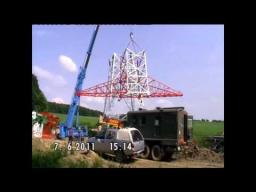Did you find an error or inaccuracy? Feel free to write us. Thank you!Tips to related online calculators
Looking for help with calculating roots of a quadratic equation?
Do you have a linear equation or system of equations and looking for its solution? Or do you have a quadratic equation?
Pythagorean theorem is the base for the right triangle calculator.

#### You need to know the following knowledge to solve this word math problem:

We encourage you to watch this tutorial video on this math problem:

## Related math problems and questions:

• Bamboo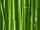Bamboo high 32 feet was at a certain height broken by the wind so the bamboo top reached the ground at a distance of 16 feet from the trunk. At what height from the ground was the bamboo broken?
• Thunderstorm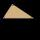The height of the pole before the storm is 10 m. After a storm when they come to check it they see that on the ground from the pole blows part of the column. Distance from the pole is 3 meters. At how high was the pole broken? (In fact, a rectangular tria
• Broken treeThe tree was 35 meters high. The tree broke at the height of 10 m above the ground. Top but does not fall off it refuted on the ground. How far from the base of the tree lay its peak?
• Broken treeThe tree is broken at 4 meters above the ground and the top of the tree touches the ground at a distance of 5 from the trunk. Calculate the original height of the tree.
• Column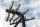Perpendicular pole high 8 m tall broke and its toe fell 2.7 m from the bottom of the pole. At what height above the ground pole broke?How long is a ladder that touches on a wall 4 meters high, and its lower part is 3 meters away from the wall?
• Acreage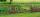What acreage has a rectangular plot whose diagonal is 34 meters long and one side has a length of 16 meters. ...
• The gardenThe garden has the shape of a rectangular trapezium. The bases have lengths of 27 meters and 36 meters, the trapezoid's height is 12 meters. Calculate how much fence will cost this garden if one meter costs 1.5 €?
• The mastThe top of the pole we see at an angle of 45°. If we approach the pole by 10 m, we see the top of the pole at an angle of 60°. What is the height of the pole?
• An observerAn observer standing west of the tower sees its top at an altitude angle of 45 degrees. After moving 50 meters to the south, he sees its top at an altitude angle of 30 degrees. How tall is the tower?
• Free space in the gardenThe grandfather's free space in the garden was in the shape of a rectangular triangle with 5 meters and 12 meters in length. He decided to divide it into two parts and the height of the hypotenuse. For the smaller part creates a rock garden, for the large
• SailboatThe 20 m long sailboat has an 8 m high mast in the middle of the deck. The top of the mast is fixed to the bow and stern with a steel cable. Determine how much cable is needed to secure the mast and what angle the cable will make with the ship's deck.
• The pole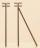The telegraph pole is supported by a 4 m bullet at 3/4 of its height, the end of which is at a distance 2.5 m from the pole post. Calculate the height of the telegraph pole.
• Billiard ballsA layer of ivory billiard balls of radius 6.35 cm is in the form of a square. The balls are arranged so that each ball is tangent to every one adjacent to it. In the spaces between sets of 4 adjacent balls other balls rest, equal in size to the original.
• A boyA boy of height 1.7m is standing 30m away from the flagstaff on the same level ground. He observes that the angle of deviation of the top of flagstaff is 30 degrees. Calculate the height of flagstaff.
• Woman's day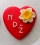We can easily make a heart for mothers for Woman's day by drawing two semicircles to the two upper sides of the square standing on their top. What is the radius of the circle circumscribed by this heart when the length of the side of the square is 1?
• The ropeA 68-centimeter long rope is used to make a rhombus on the ground. The distance between a pair of opposite side corners is 16 centimeters. What is the distance between the other two corners?